# Tamilnadu Board Class 10 Maths Solutions Chapter 1 Relations and Functions Unit Exercise 1

## Tamilnadu State Board Class 10 Maths Solutions Chapter 1 Relations and Functions Unit Exercise 1

Question 1.
If the ordered pairs (x2 – 3x,y2 + 4y) and (-2,5) are equal, then find x and y.
Solution:
(x2 – 3x, y2 + 4y) = (-2,5)
x2 – 3x = -2
x2 – 3x + 2 = 0
(x – 2)(x – 1) = 0Question 2.
The Cartesian product A × A has 9 elements among which (-1,0) and (0,1) are found. Find the set A and the remaining elements of A×A.
Solution:
A = {-1,0, 1},B = {1,0,-1}
A × B = {(-1,1), (-1,0), (-1,-1), (0,1), (0, 0), (0,-1), (1,1), (1,0), (1,-1)}

Question 3.
Given that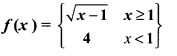(i) f(0)
(ii) f(3)
(iii) f(a + 1) in terms of a.(Given that a > 0)
Solution:
(i) f(0) = 4
(ii) f(3) = $$\sqrt { 3-1 }$$ = $$\sqrt { 2 }$$
(iii) f(a+ 1) = $$\sqrt { a+1-1 }$$ = $$\sqrt { a }$$

Question 4.
Let A = {9,1O,11,12,13,14,15,16,17} and let f: A → N be defined by f(n) = the highest prime factor of n ∈ A. Write f as a set of ordered pairs and find the range of f.
Solution:
A = {9,10,11, 12, 13, 14,15,16,17}
f: A → N
f(n) = the highest prime factor of n ∈ A
f = {(9, 3), (10, 5), (11, 11), (12, 3), (13,13), (14, 7), (15, 5), (16,2), (17, 17)}
Range = {3,5, 11, 13,7,2, 17}
= {2,3,5,7,11,13,17}

Question 5.
Find the domain of the function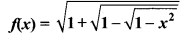Solution:Question 6.
If f(x)= x2, g(x) = 3x and h(x) = x – 2 Prove that (fog)oh = fo(goh).
Solution:
f(x) = x2
g(x) = 3x
h(x) = x – 2
(fog)oh = x – 2
LHS = fo(goh)
fog = f(g(x)) = f(3x) = (3x)2 = 9x2
(fog)oh = (fog) h(x) = (fog) (x – 2)
= 9(x – 2)2 = 9(x2 – 4x + 4)
= 9x2 – 36x + 36 …..(1)
RHS = fo(goh)
(goh) = g(h(x)) = g(x- 2)
= 3(x – 2) = 3x – 6
fo(goh) = f(3x – 6) = (3x – 6)2
= 9x2 – 36x + 36 ……(2)
(1) = (2)
LHS = RHS
(fog)oh = fo(goh) is proved.

Question 7.
A = {1, 2} and B = {1, 2, 3, 4} , C = {5, 6} and D = {5, 6, 7, 8} . Verify whether A × C is a subset of B × D?
Solution:
A = {1,2),B = (1,2,3,4)
C = {5,6},D = {5,6,7,8)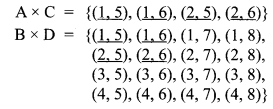(A × C) ⊂ (B × D) It is proved.

Question 8.
If f(x) =$$\frac { x-1 }{ x+1 }$$, x ≠ 1 show that f(f(x)) = – $$\frac { 1 }{ x }$$, Provided x ≠ 0.
Solution:Hence it is proved.

Question 9.
The function/and g are defined by f(x) = 6x + 8; g(x) = $$\frac { x-2 }{ 3 }$$.
(i) Calculate the value of gg ($$\frac { 1 }{ 2 }$$)
(ii) Write an expression for g f(x) in its simplest form.
Solution: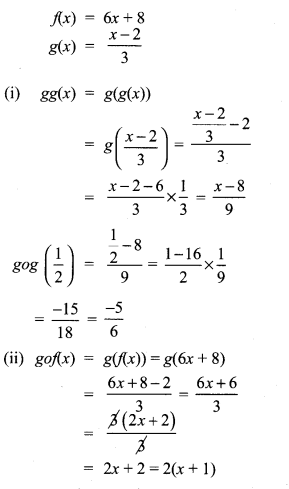Question 10.
Write the domain of the following real functions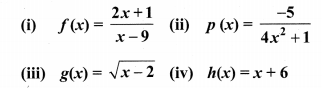Solution:
(i) f(x) = $$\frac { 2x+1 }{ x-9 }$$
The denominator should not be zero as the function is a real function.
∴ The domain = R – {9}
(ii) p(x) = $$\frac{-5}{4 x^{2}+1}$$
The domain is R.
(iii) g(x) = $$\sqrt { x-2 }$$
The domain = (2, ∝)
(iv) h(x) = x + 6
The domain is R.